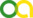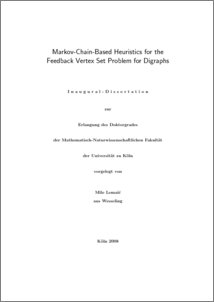Lemaic, Mile (2008). Markov-Chain-Based Heuristics for the Feedback Vertex Set Problem for Digraphs. PhD thesis, Universität zu Köln.Preview
PDF
Dissertation.pdf

## Abstract

A feedback vertex set (FVS) of an undirected or directed graph G=(V, A) is a set F such that G-F is acyclic. The minimum feedback vertex set problem asks for a FVS of G of minimum cardinality whereas the weighted minimum feedback vertex set problem consists of determining a FVS F of minimum weight w(F) given a real-valued weight function w. Both problems are NP-hard [Karp72]. Nethertheless, they have been found to have applications in many fields. So one is naturally interested in approximation algorithms. While most of the existing approximation algorithms for feedback vertex set problems rely on local properties of G only, this thesis explores strategies that use global information about G in order to determine good solutions. The pioneering work in this direction has been initiated by Speckenmeyer [Speckenmeyer89]. He demonstrated the use of Markov chains for determining low cardinality FVSs. Based on his ideas, new approximation algorithms are developed for both the unweighted and the weighted minimum feedback vertex set problem for digraphs. According to the experimental results presented in this thesis, these new algorithms outperform all other existing approximation algorithms. An additional contribution, not related to Markov chains, is the identification of a new class of digraphs G=(V, A) which permit the determination of an optimum FVS in time O(|V|^4). This class strictly encompasses the completely contractible graphs [Levy/Low88].

Item Type: Thesis (PhD thesis)
Translated title:
TitleLanguage
Markov-Ketten-Basierte Heuristiken für das Feedback Vertex Set Problem für DigraphenGerman
Creators:
CreatorsEmailORCID
Lemaic, Milelemaic@uni-koeln.deUNSPECIFIED
URN: urn:nbn:de:hbz:38-25472
Subjects: Data processing Computer science
Faculty: Faculty of Mathematics and Natural Sciences
Divisions: Faculty of Mathematics and Natural Sciences > Department of Mathematics and Computer Science > Institute of Computer Science
Language: English
Date: 2008
Date of oral exam: 29 June 2008
Referee:View Item